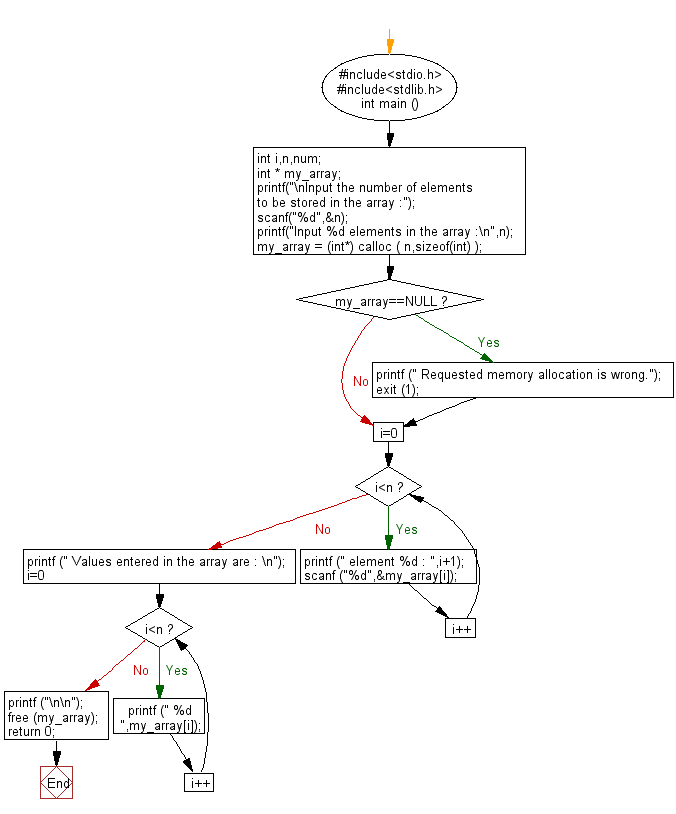﻿ C Program: Allocate a block of memory for an array - w3resource

# C Exercises: Allocate a block of memory for an array

## C Variable Type : Exercise-11 with Solution

Write a C program to allocate a block of memory for an array.

Sample Solution:

C Code:

``````#include<stdio.h>      // Include the standard input/output header file.
#include<stdlib.h>     // Include the standard library header file.

int main ()          // Start of the main function.
{
int i, n, num;    // Declare integer variables 'i', 'n', and 'num'.
int * my_array;   // Declare a pointer to int 'my_array'.

printf("\nInput the number of elements to be stored in the array :");   // Prompt the user to input the number of elements.
scanf("%d",&n);   // Read the user's input and store it in 'n'.

printf("Input %d elements in the array :\n",n);   // Prompt the user to input the elements of the array.

my_array = (int*) calloc( n,sizeof(int) );   // Allocate memory for 'n' integers and assign the pointer to 'my_array'.
if (my_array==NULL)   // Check if memory allocation was successful.
{
printf (" Requested memory allocation is wrong.");   // Print an error message if memory allocation failed.
exit (1);   // Exit the program with an error code.
}

for ( i=0; i<n; i++ )   // Start of a for loop to iterate over the elements of the array.
{
printf (" element %d : ",i+1);   // Prompt the user for the value of the current element.
scanf ("%d",&my_array[i]);     // Read the user's input and store it in the current element of 'my_array'.
}

printf (" Values entered in the array are : \n");   // Print a message indicating the values in the array.
for ( i=0; i<n; i++ )   // Start of a for loop to iterate over the elements of the array.
printf (" %d ",my_array[i]);   // Print the current element of the array.

printf ("\n\n");   // Print two newline characters for formatting.

free (my_array);   // Free the allocated memory for 'my_array'.

return 0;   // Return 0 to indicate successful execution of the program.
}   // End of the main function.
```
```

Sample Output:

```Input the number of elements to be stored in the array :5
Input 5 elements in the array :
element 1 : 5
element 2 : 10
element 3 : 15
element 4 : 20
element 5 : 25
Values entered in the array are :
5  10  15  20  25
```

Flowchart:C Programming Code Editor:

What is the difficulty level of this exercise?

Test your Programming skills with w3resource's quiz.

﻿